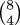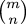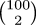# The Universe of Discourse

Wed, 13 Jun 2007

How to calculate binomial coefficients, again
Yesterday's article about how to calculate binomial coefficients was well-received. It was posted on Reddit, and to my surprise and gratification, the comments were reasonably intelligent. Usually when a math article of mine shows up on Reddit, all the megacretins come out of the woodwork to say what an idiot I am, and why don't I go back to school and learn basic logic.

A couple of people pointed out that, contrary to what I asserted, the algorithm I described can in fact overflow even when the final result is small enough to fit in a machine word. Considerfor example. The algorithm, as I wrote it, calculates intermediate values 8, 8, 56, 28, 168, 56, 280, 70, and 70 is the final answer. If your computer has 7-bit machine integers, the answer (70) will fit, but the calculation will overflow along the way at the 168 and 280 steps.

Perhaps more concretely, !!35\choose11!! is 417,225,900, which is small enough to fit in a 32-bit unsigned integer, but the algorithm I wrote wants to calculate this as !!35{34\choose10}\over11!!, and the numerator here is 4,589,484,900, which does not fit.

One Reddit user suggested that you can get around this as follows: To multiply r by a/b, first check if b divides r. If so, calculate (r/ba; otherwise calculate (r·a)/b. This should avoid both overflow and fractions.

Unfortunately, it does not. A simple example is !!{14\choose4} = {11\over1}{12\over2}{13\over3}{14\over4}!!. After the first three multiplications one has 286. One then wants to multiply by 14/4. 4 does not divide 286, so the suggestion calls for multiplying 286 by 14/4. But 14/4 is 3.5, a non-integer, and the goal was to use integer arithmetic throughout.

 BuyThe Art of Computer Programming: Volume 2, Seminumerical Algorithmsfrom Bookshop.org(with kickback)(without kickback)
Fortunately, this is not hard to fix. Say we want to multiply r by a/b without overflow or fractions. First let g be the greatest common divisor of r and b. Then calculate ((r/g) · a)/(b/g). In the example above, g is 2, and we calculate (286/2) · (14/2) = 143 · 7; this is the best we can do.

I haven't looked, but it is hard to imagine that Volume II of Knuth doesn't discuss this in exhaustive detail, including all the stuff I just said, plus a bunch of considerations that hadn't occurred to any of us.

A few people also pointed out that you can save time when n > m/2 by calculating !!m\choose m-n!! instead of. For example, instead of calculating !!100\choose98!!, calculate. I didn't mention this in the original article because it was irrelevant to the main point, and because I thought it was obvious.